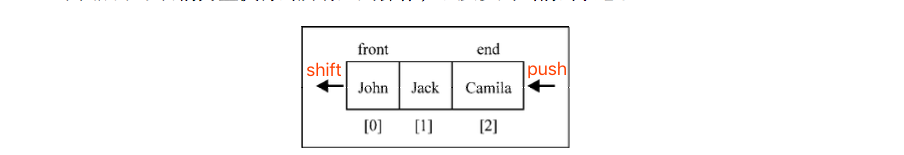# 《学习JavaScript数据结构与算法》笔记

@一棵菜菜  July 16, 2019

# 第二章 数组

## 使用map和filter方法

`map``filter`遍历数组，会返回新数组的遍历方法。

## reduce

``````function total(array) {
return array.reduce((prev, current, index, array) => {
return prev + current;
}, 0);
}

const data = total([1, 2, 3, 4, 5]); // 15``````

## 2.7.3 ECMAScript 6 和数组的新功能 【p42】

`Array.from()`：根据已有的数组创建一个新数组。

``````let numbers = [1,2];
let numbers2 = Array.from(numbers);

// 过滤值的函数
let numbers3 = Array.from(numbers, x => return x % 2 == 0);``````

`Array.of()`：根据传入的参数创建一个新数组。

``````let numbers3 = Array.of(1); // 
let numbers4 = Array.of(1, 2, 3); // [1, 2, 3]``````

`Array.fill()`：用静态值填充数组。

``let ones = Array(3).fill(1); // [1,1,1]``

`includes`:如果数组中存在某个元素则返回true，否则返回false。ES7新增。
`find`：根据回调函数给定的条件从数组中查找元素，返回通过测试（函数内判断）的数组的第一个元素的值。
`findIndex`：根据回调函数给定的条件从数组中查找元素，返回传入一个测试条件（函数）符合条件的数组第一个元素位置。

``````var a = [1,2,3,4];
a.includes(3); // true
let b = a.find((x)=>{return x>2});b; // 3
let c = a.findIndex((x)=>{return x>2});c; // 2``````

# 第三章 栈

### 数据结构

• 数组（计算机科学中最常用的数据结构）
• 队列

## 栈1. 栈也被用在编程语言的编译器和内存中保存变量、方法调用等。—— js的执行栈（event loop）
2. 可以存储访问过的任务或路径、撤销的操作
3. Java和C#用栈来存储变量和方 法调用，特别是处理递归算法时，有可能抛出一个栈溢出异常。

### 向栈添加元素

push方法负责往栈里添加新元素。

【牢记】该方法只添加元素到栈顶，也就是栈的末尾

### 从栈移除元素

pop方法。

【牢记】移出的是最后添加进去的元素

### 实现栈

``````
/**
* 栈
*/
let Stack = (function() {
let _items = Symbol("items");
class Stack {
constructor() {
this[_items] = [];
}

// 添加一个(或几个)新元素到栈顶
push(item) {
// 创建了一个假的私有属性（因可以通过Object.getOwnPropertySymbols()获取到Symbol属性）
this[_items].push(item);
}

// 移除栈顶的元素，同时返回被移除的元素。
pop() {
return this[_items].pop();
}

// 返回栈顶的元素，不对栈做任何修改
peek() {
return this[_items][this.size() - 1];
}

size() {
return this[_items].length;
}

isEmpty() {
return this.size() === 0;
}

clear() {
this[_items] = [];
}

print() {
console.log(this[_items].toString());
}
}

return Stack;
})();

let stack = new Stack();
stack.push(5);

let symbols = Object.getOwnPropertySymbols(stack); // [Symbol(items)]
console.log(stack[symbols]);``````

### 用栈解决问题

• 十进制转二进制问题，以及任意进制转换的算法;
• 平衡圆括号问题;【重点】
• 如何用栈解决汉诺塔问题。【重点】
``````var isValid = function(string) {
const arr = string.split("");

let map = {
"(": -1,
")": 1,
"[": -2,
"]": 2,
"{": -3,
"}": 3
};

let stack = [];
for (let i = 0; i < arr.length; i++) {
if (map[arr[i]] < 0) {
stack.push(arr[i]);
} else {
let stackLast = stack.pop();
if (map[arr[i]] + map[stackLast] !== 0) {
return false;
}
}
}

if (stack.length > 0) {
return false;
}

return true;
};

// stack里保存的都是左括号（值<0）
// 规律：遍历的当前一个如果是正数，则判断与栈中的最后一个相加是否为0，不为0则是false。为0时又已经从stack中移除了，所以不影响下一个的判断。

let result = isValid("[[]{}()]");
console.log(result); // true``````

## 队列### 实现队列

``````class Queue {
constructor() {
this.items = [];
}

// 入队：向队列尾部添加一个(或多个)新的项
enQueue(item) {
this.items.push(item);
}

// 出队：移除队列的第一(即排在队列最前面的)项，并返回被移除的元素
deQueue() {
return this.items.shift();
}

// 查看队头元素
return this.items;
}

// 查看队列里元素个数
getSize() {
return this.items.length;
}

// 判断队列是否为空
isEmpty() {
return this.getLength() === 0;
}

// 清空队列
clear() {
this.items = [];
}

print() {
console.log(this.items.toString());
}
}

var queue = new Queue();``````